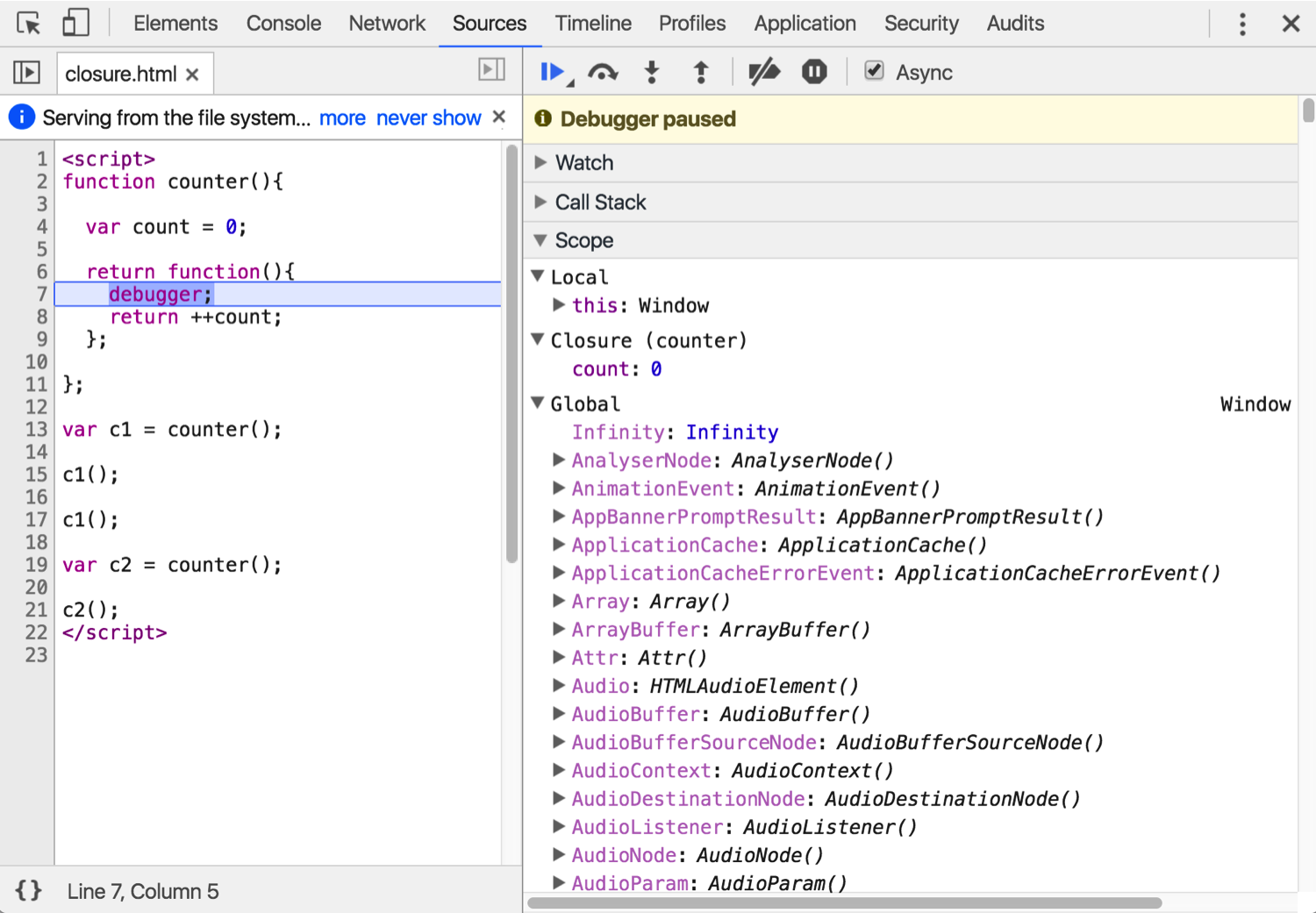# Scope `page`

Learn how to see the variables available in the scope.

## The problem

What is the value of `VARIABLE_IN_SCOPE` in the following code?

``````<script src="//bitovi.github.io/academy/static/scripts/debugging/variables.js"></script>
<script type="module">
// What is the value of VARIABLE_IN_SCOPE?
function a(){
var a = makeAVariables;
return function b(){
var VARIABLE_IN_SCOPE = makeBVariables;
return function c(){
var c = makeCVariables;
return function d(){
var d = makeDVariables;
console.log("What is the value of VARIABLE_IN_SCOPE?");
debugger;
eval("");
};
};
};
}
a()()()();
</script>
``````

## What you need to know

Chrome's developer tools allow you to inspect variables in the scope. For example, consider the following code from the Closures training:

``````function counter(){

var count = 0;

return function(){
debugger;
return ++count;
};

};

var c1 = counter();

c1();

c1();

var c2 = counter();

c2();
``````

Chrome's developers tools allows you to inspect variables in the scope as follows:## The solution

The answer is `d`.